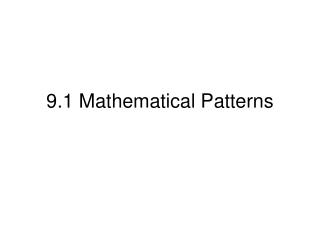DownloadDownload Presentation9.1 Mathematical Patterns

# 9.1 Mathematical Patterns

Download Presentation## 9.1 Mathematical Patterns

- - - - - - - - - - - - - - - - - - - - - - - - - - - E N D - - - - - - - - - - - - - - - - - - - - - - - - - - -
##### Presentation Transcript

1. 9.1 Mathematical Patterns

2. Sometimes steps in a process form a pattern. You can describe some patterns with a sequence, or ordered list of numbers. Each number in a sequence is a term.

3. Example: Suppose you drop a ball from a height of 10 feet, after each time it hits the ground it rebounds 85% of its previous height. How high will it rebound on its fourth bounce? 10ft bounce (.85) * 10 8.5 ft bounce (.85) * 8.5 7.225 ft bounce (.85) * 7.225 6.141 ft bounce (.85) * 6.141 5.220 feet on 4th bounce

4. We can use a variable, such as a, with a positive integer subscript to represent the terms in a sequence. 1st term 2nd term 3rd term a1 a2 a3 … n-1 term nth term n+1 term an-1 an an+1

5. A recursive formula defines the terms in a sequence by relating them to the term before. You always give the initial value. The first example was recursive because the next height was related to the previous height. The formula for example one is: an = 0.85an-1 where a1 = 10

6. A formula that expresses the nth term in terms of n is an explicit formula. Example: Write an explicit formula for each sequence. Find a12. 4,5,6,7,8,…. The formula is an = n+3 a12 = 15 Write a recursive formula? an = an-1 +1 a1 = 4

7. CW pg 569 7 – 14, 15 – 35 odds